##### The Japanese Bonsai specialist
Direct order Contact Help / Services Newsletter# Ophiopogon japonicus minor

Ophiopogon japonicus minor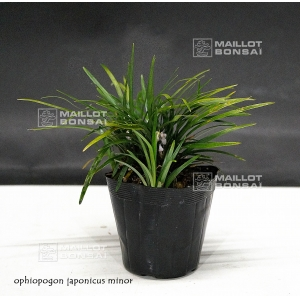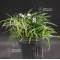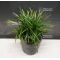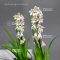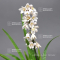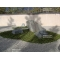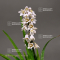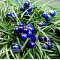ref. : 9999

5,00

Available quantity : 2Order

###### Description

Perennial plant with evergreen leaves of 3/6 cm large and 0.2 width of a dark green. Flowering in summer with its pink-toned white bunches that give birth to fruits, similar to bright purple blueberries.

Tuft delivered in a small and flexible plastic pot, diameter or the small pot : 6 cm. Strong and dense tuft coming from dividing during its third cultivation year.

Uncommon plant with bright leaves, used as well kusamono as flower bed in garden. Winter protection in very cold regions, in case of snow during winter the foliage becomes brown but grown again in spring. This species is usually very used for the making of japanese garden’s flower beds.

Last picture japanese garden scene for information.

#small 4.5 #kusamono 3.8 #garden 3.6 #with 3.4 #ophiopogon 3 #japonicus 2.9 #japanese 2.8 #during 2.6 #plants 2.6 #flower 2.6

Formule
(( ROUND((CHAR_LENGTH(b.article_nom)-CHAR_LENGTH(REPLACE(b.article_nom, 'garden', '')))/LENGTH('garden')) + ROUND((CHAR_LENGTH(b.article_description)-CHAR_LENGTH(REPLACE(b.article_description, 'garden', '')))/LENGTH('garden')) ) * 3.6) + (( ROUND((CHAR_LENGTH(b.article_nom)-CHAR_LENGTH(REPLACE(b.article_nom, 'with', '')))/LENGTH('with')) + ROUND((CHAR_LENGTH(b.article_description)-CHAR_LENGTH(REPLACE(b.article_description, 'with', '')))/LENGTH('with')) ) * 3.4) + (( ROUND((CHAR_LENGTH(b.article_nom)-CHAR_LENGTH(REPLACE(b.article_nom, 'ophiopogon', '')))/LENGTH('ophiopogon')) + ROUND((CHAR_LENGTH(b.article_description)-CHAR_LENGTH(REPLACE(b.article_description, 'ophiopogon', '')))/LENGTH('ophiopogon')) ) * 3) + (( ROUND((CHAR_LENGTH(b.article_nom)-CHAR_LENGTH(REPLACE(b.article_nom, 'japonicus', '')))/LENGTH('japonicus')) + ROUND((CHAR_LENGTH(b.article_description)-CHAR_LENGTH(REPLACE(b.article_description, 'japonicus', '')))/LENGTH('japonicus')) ) * 2.9) + (( ROUND((CHAR_LENGTH(b.article_nom)-CHAR_LENGTH(REPLACE(b.article_nom, 'japanese', '')))/LENGTH('japanese')) + ROUND((CHAR_LENGTH(b.article_description)-CHAR_LENGTH(REPLACE(b.article_description, 'japanese', '')))/LENGTH('japanese')) ) * 2.8) + (( ROUND((CHAR_LENGTH(b.article_nom)-CHAR_LENGTH(REPLACE(b.article_nom, 'leaves', '')))/LENGTH('leaves')) + ROUND((CHAR_LENGTH(b.article_description)-CHAR_LENGTH(REPLACE(b.article_description, 'leaves', '')))/LENGTH('leaves')) ) * 2.6) + (( ROUND((CHAR_LENGTH(b.article_nom)-CHAR_LENGTH(REPLACE(b.article_nom, 'flower', '')))/LENGTH('flower')) + ROUND((CHAR_LENGTH(b.article_description)-CHAR_LENGTH(REPLACE(b.article_description, 'flower', '')))/LENGTH('flower')) ) * 2.6) + (( ROUND((CHAR_LENGTH(b.article_nom)-CHAR_LENGTH(REPLACE(b.article_nom, 'during', '')))/LENGTH('during')) + ROUND((CHAR_LENGTH(b.article_description)-CHAR_LENGTH(REPLACE(b.article_description, 'during', '')))/LENGTH('during')) ) * 2.6) + (( ROUND((CHAR_LENGTH(b.article_nom)-CHAR_LENGTH(REPLACE(b.article_nom, 'bright', '')))/LENGTH('bright')) + ROUND((CHAR_LENGTH(b.article_description)-CHAR_LENGTH(REPLACE(b.article_description, 'bright', '')))/LENGTH('bright')) ) * 2.6) + (( ROUND((CHAR_LENGTH(b.article_nom)-CHAR_LENGTH(REPLACE(b.article_nom, 'winter', '')))/LENGTH('winter')) + ROUND((CHAR_LENGTH(b.article_description)-CHAR_LENGTH(REPLACE(b.article_description, 'winter', '')))/LENGTH('winter')) ) * 2.6)

## Secure payment## Delivery

Our logistic partners :